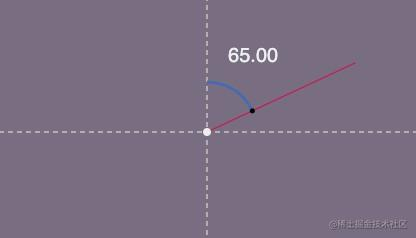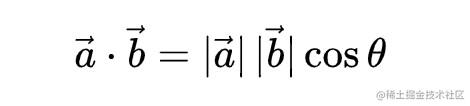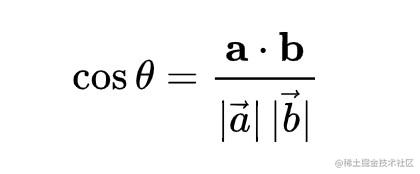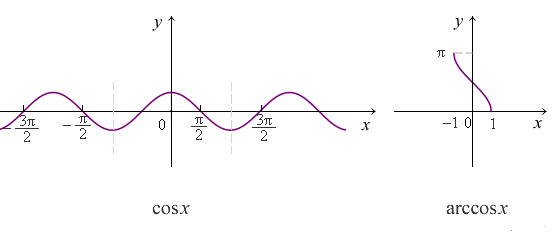求向量的角度

问题描述计算function calCos(a, b) {
// 点积
let dotProduct = a * b + a * b;
let d = Math.sqrt(a * a + a * a) * Math.sqrt(b * b + b * b);
return dotProduct/d;
}

let angle = Math.acos(radian) * 180 / Math.PI;let angle = Math.acos(radian) * 180 / Math.PI;
if (x < cx) angle = -angle;

完整代码

function calAngle(cx, cy, x, y) {
const radian = getCosBy2pt(x, y, cx, cy);
let angle = Math.acos(radian) * 180 / Math.PI;

if (x < cx) angle = -angle;
return angle;

// 计算 点1指点2形成 的向量
function getCosBy2pt(x, y, cx, cy) {
let a = [x - cx, y - cy];
let b = [0, -1];
return calCos(a, b);
}
function calCos(a, b) {
// 点积
let dotProduct = a * b + a * b;
let d = Math.sqrt(a * a + a * a) * Math.sqrt(b * b + b * b);
return dotProduct/d;
}
}

演示

codepen.io/F-star/pen/…